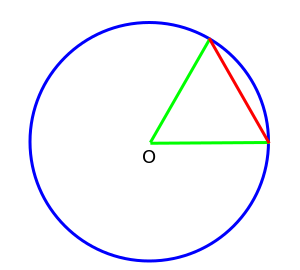# Degree (angle)

A degree (in full, a degree of arc, arc degree, or arcdegree), usually denoted by ° (the degree symbol), is a measurement of plane angle, representing 1360 of a full rotation; one degree is equivalent to π/180 radians. When that angle is with respect to a reference meridian, it indicates a location along a great circle of a sphere, such as Earth (see Geographic coordinate system), Mars, or the celestial sphere.

## HistoryA circle with an equilateral Chord (geometry) (red). One sixtieth (1/60) of this arc is a degree. Six such chords complete the circle.

The selection of 360 as the number of degrees (i.e., smallest practical sub-arcs) in a circle was probably based on the fact that 360 is approximately the number of days in a year. Its use is often said to originate from the methods of the ancient Babylonians. Ancient astronomers noticed that the stars in the sky, which circle the celestial pole every day, seem to advance in that circle by approximately one-360th of a circle, i.e., one degree, each day. (Primitive calendars, such as the Persian Calendar, used 360 days for a year.) Its application to measuring angles in geometry can possibly be traced to Thales who popularized geometry among the Greeks and lived in Anatolia (modern western Turkey) among people who had dealings with Egypt and Babylon.

The earliest trigonometry, used by the Babylonian astronomers and their Greek successors, was based on chords of a circle. A chord of length equal to the radius made a natural base quantity. One sixtieth of this, using their standard sexagesimal divisions, was a degree; while six such chords completed the full circle.

Another motivation for choosing the number 360 is that it is readily divisible: 360 has 24 divisors (including 1 and 360), including every number from 1 to 10 except 7. For the number of degrees in a circle to be divisible by every number from 1 to 10, there would need to be 2520 degrees in a circle, which is a much less convenient number.

Divisors of 360 are 1, 2, 3, 4, 5, 6, 8, 9, 10, 12, 15, 18, 20, 24, 30, 36, 40, 45, 60, 72, 90, 120, 180, and 360.

### India

The division of the circle into 360 parts also occurred in ancient India, as evidenced in the Rig Veda:

Twelve spokes, one wheel, navels three.
Who can comprehend this?
On it are placed together
three hundred and sixty like pegs.
They shake not in the least.
(Dirghatama, Rig Veda 1.164.48)

## Subdivisions

For many practical purposes, a degree is a small enough angle that whole degrees provide sufficient precision. When this is not the case, as in astronomy or for latitudes and longitudes on the Earth, degree measurements may be written with decimal places, but the traditional sexagesimal unit subdivision is commonly seen. One degree is divided into 60 minutes (of arc), and one minute into 60 seconds (of arc). These units, also called the arcminute and arcsecond, are respectively represented as a single and double prime, or if necessary by a single and double quotation mark: for example, 40.1875° = 40° 11′ 15″ (or 40° 11' 15").

If still more accuracy is required, decimal divisions of the second are normally used, rather than thirds of 160 second, fourths of 160 of a third, and so on. These (rarely used) subdivisions were noted by writing the Roman numeral for the number of sixtieths in superscript: 1I for a "prime" (minute of arc), 1II for a second, 1III for a third, 1IV for a fourth, etc. Hence the modern symbols for the minute and second of arc.

## Alternative units

In most mathematical work beyond practical geometry, angles are typically measured in radians rather than degrees. This is for a variety of reasons; for example, the trigonometric functions have simpler and more "natural" properties when their arguments are expressed in radians. These considerations outweigh the convenient divisibility of the number 360. One complete circle (360°) is equal to 2π radians, so 180° is equal to π radians, or equivalently, the degree is a mathematical constant ° = π180.

With the invention of the metric system, based on powers of ten, there was an attempt to define a "decimal degree" (grad or gon), so that the number of decimal degrees in a right angle would be 100 gon, and there would be 400 gon in a circle. This idea, however, did not gain much momentum.

An angular mil which is most used in military applications has at least three specific variants.

In computer games which depict a three-dimensional virtual world, the need for very fast computations resulted in the adoption of a binary, 256 degree system. In this system, a right angle is 64 degrees, angles can be represented in a single byte, and all trigonometric functions are implemented as small lookup tables. These units are sometimes called "binary radians" ("brads") or "binary degrees."